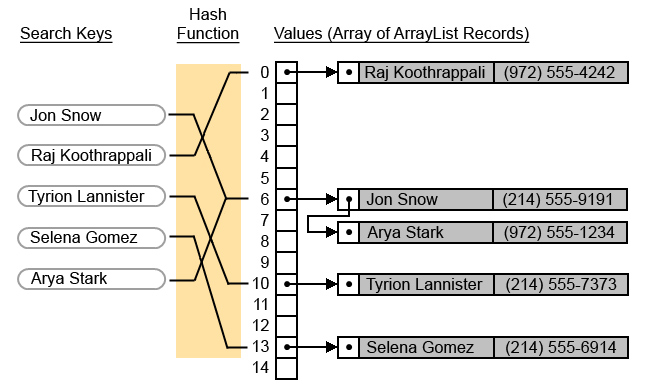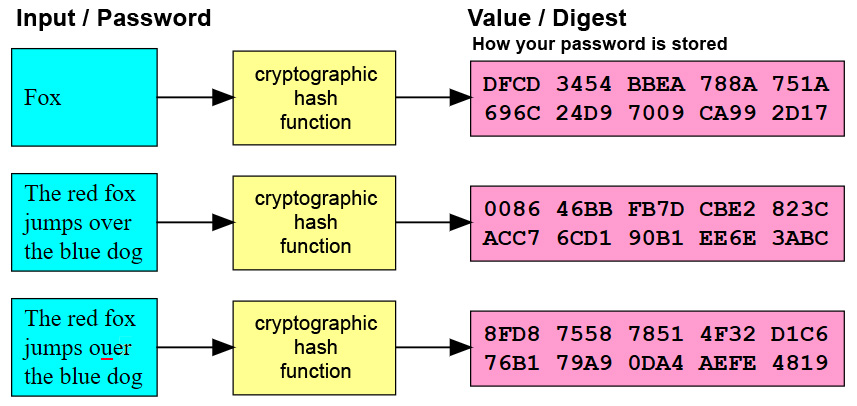Java Chapter 17 - Hashing

17.1 Hash Table

A hash table is a method of storing data that improves the searching efficiency to O(1).  This means that the size of the data set (n) has no effect on the length of time it takes to search.  The hash table has two parts: (1) the array of data, and (2) a hash function that maps each data item to an index in the array.  The diagram below shows a 15 element array to store the values.  When you search for a name (search keys), a hash function maps it to an index in the array.An example hash function could convert text keys to numbers (e.g. add the ASCII values for each character).  Once you get a number for the text, you could mod it by the data set size (15) to get the index for where to store the value.  This type of hash function will give you an index within the range of your array size, but does not guarantee that the index will be unique.  Therefore, you will have collisions where multiple values map to the same index number.  There are many ways to handle collisions.  One way is to keep an ArrayList to hold all the keys that map to the same index (e.g. Jon Snow and Arya Stark).

 Search Key Hash Function Converts Text to Number Mod by data set size (15) to get Index Jon Snow 201 201 % 15 = 6 Raj Koothrappali 540 540 % 15 = 0 Tyrion Lannister 310 310 % 15 = 10 Selena Gomez 268 268 % 15 = 13 Arya Stark 186 186 % 15 = 6

17.2 Cryptographic Hashing

Passwords on servers are usually stored as cryptographic hashes.  A cryptographic hash function has these properties:

(1)  It is very difficult to reverse. The hash function converts the Input into a digest (a summarized form), and is therefore considered to be a one way function.
(2)  It creates a fixed-size alphanumeric string, or hash value.
(3)  Small changes in the source input drastically change the hashed value.
(4)  Collisions (two different inputs that create the same hash value) are possible, but statistically are rare.Popular cryptographic hash functions include MDA and SHA.  Hackers have created huge precomputed lookup or "rainbow tables" for character combinations.  They can run the has values through the table to see if it matches a password.  To make this even more difficult, hashes are usually "salted".  One way to salt the hash is to add a large random number to a user's password before it is hashed.  The hacker would have to generate a new rainbow table for each user.

17.3 Hashtable Class

The Hashtable class is part of java.util.  It stores key/value pairs in a hash table.  Below are a few of the methods.

 Method Description clear() Clears and resets the hash table. get(Object key) Returns the value of the specified key.  If the key is not in the hash table, then it returns null. isEmpty() Returns true if the hash table has no key/value pairs. put(Object key, Object value) Inserts a key/value pair into the hash table. remove(Object key) Removes the key/value pair from the hash table. size() Returns the number of entries in the hash table.

The program below demonstrates a simple hash table.

 hash.java import java.util.*; public class hash {    public static void main(String[] args)    {       // Create Hashtable       Hashtable MyHTable = new Hashtable();       // Add Key and Value pairs to MyHTable       MyHTable.put("apple",73);       MyHTable.put("grape",42);       MyHTable.put("kiwi",69);       System.out.println(MyHTable.get("grape"));    } } Output 42